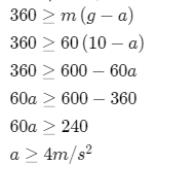Courses

# Newton's Law Of Motion NAT Level - 1

## 10 Questions MCQ Test Basic Physics for IIT JAM | Newton's Law Of Motion NAT Level - 1

Description
This mock test of Newton's Law Of Motion NAT Level - 1 for Physics helps you for every Physics entrance exam. This contains 10 Multiple Choice Questions for Physics Newton's Law Of Motion NAT Level - 1 (mcq) to study with solutions a complete question bank. The solved questions answers in this Newton's Law Of Motion NAT Level - 1 quiz give you a good mix of easy questions and tough questions. Physics students definitely take this Newton's Law Of Motion NAT Level - 1 exercise for a better result in the exam. You can find other Newton's Law Of Motion NAT Level - 1 extra questions, long questions & short questions for Physics on EduRev as well by searching above.
*Answer can only contain numeric values
QUESTION: 1

### Figure shows two pulley arrangements for lifting a mass m.  In (a) the mass is lifted by attaching a mass 2m.  While in (b) the mass is filled by pulling the other end with a downward force F = 2mg.  If  fa and fb are the accelerations of the two masses such that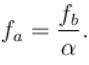Find the value of  α (Assume string is massless and pulley is ideal)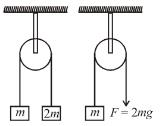.

Solution: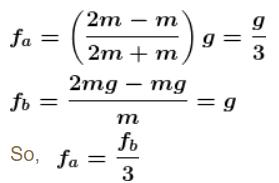*Answer can only contain numeric values
QUESTION: 2

### Figure shows a 5kg ladder hanging from a string that is connected with a ceiling and is having a spring balance connected in between. A boy of mass 25kg is climbing up the ladder at acceleration 1m/s2.  Assuming the spring balance and the string to be massless and the spring to show a constant reading the reading to the spring balance ( in kg ) is...........  Take  g = 10m/s2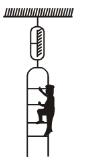Solution:

If reading of spring balance is T, then applying NLM on (man + ladder) system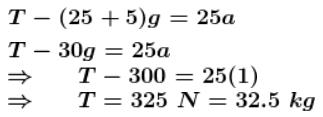*Answer can only contain numeric values
QUESTION: 3

### A man of mass m stands on a platform of equal mass m and pulls himself by two ropes passing over pulleys as shown. If he pulls each rope with a force  half of his weight, his upward acceleration would be (in m/s2)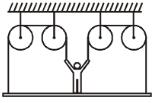Solution:

Upwards: mg/2 + mg/2 + mg/2 + mg/2
Downwards: mg + mg
Net 0 = ma => a = 0.

*Answer can only contain numeric values
QUESTION: 4

In the system shown in figure  mA = 4m, mB = 3m and m= 8m. Friction is absent everywhere. String is light and inextensible. If the system is released from rest find the acceleration ( in m/s2) of block B. ( Take  = 10 m/s2)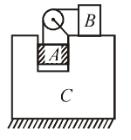Solution: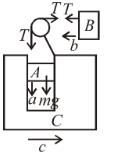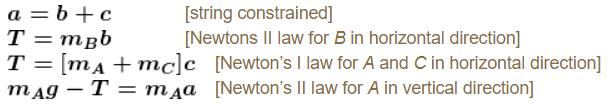*Answer can only contain numeric values
QUESTION: 5

Three blocks AB and C of mass 10kg each are hanging on a string passing over a fixed frictionless pulley as shown in figure. The tension (in Newton) in the string connecting blocks B and C is .........  Take =10 m/s2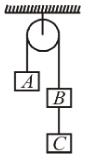Solution: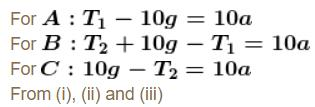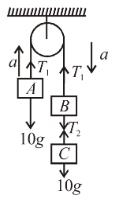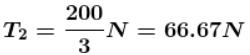*Answer can only contain numeric values
QUESTION: 6

There is an inclined surface of inclination θ = 30º. A smooth groove is cut into it forming angle α with AB.  A steel ball is free to slide along the groove. If the ball is released from the point O at top end of the groove, the speed when it when it comes to A in m/s is ...........  Take g = 10 m/s2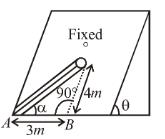Solution:

Length of groove =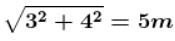acceleration along the incline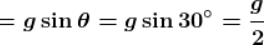acceleration along the groove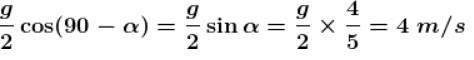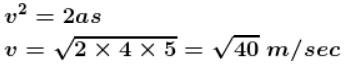*Answer can only contain numeric values
QUESTION: 7

Two blocks A and B  with mass 4kg and 6kg respectively are connected by a stretched spring of negligible mass as in figure. When the two block are released simultaneously the initial acceleration of B  is 1.5m/s2 westward. The acceleration of  A (in m/s2) is: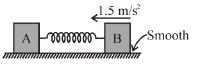Solution: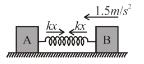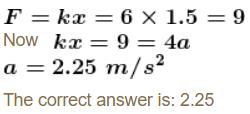*Answer can only contain numeric values
QUESTION: 8

Consider the system as shown in the figure. The pulley and the string are light and all the surfaces are frictionless. The tension (in Newton) in the string is ............ ( g = 10m/s2 )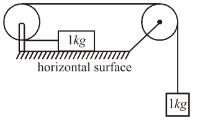Solution:

The acceleration of block of mass m1 is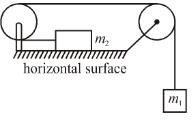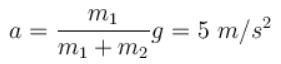From FBD of block m,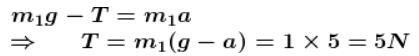QUESTION: 9

A body of mass 3kg hits a wall at an angle of 60° and with speed of 10m/s and returns at the same angle. The impact time is 0.2sec. Calculate force (in Newton)  exerted on the wall :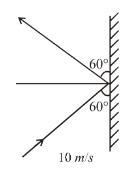Solution: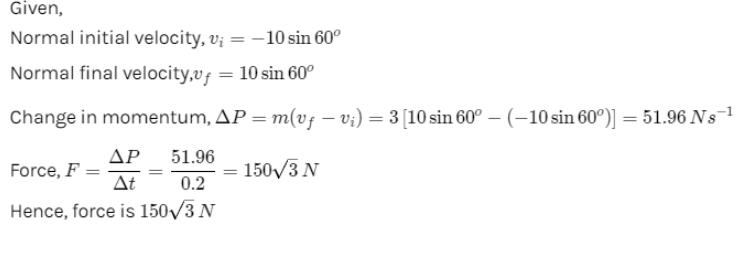*Answer can only contain numeric values
QUESTION: 10

One end of massless rope, which passes over a massless and frictionless pulley P is tied to a hook C while the other end is free. Maximum vertical force that the clamp can bear is 180N. With what value of minimum safe acceleration (in ms–2) can a man of 60kg climb down the rope?

Solution:

If man move downward with acceleration a then its apparent weight deceases. In that condition tension in string m(g — a) this should not be exceed over breaking strength of the rope i.e.